Question

Free-energy change, ΔG∘, is related to cell potential, E∘, by the equation

ΔG∘=−nFE∘

where n is the number of moles of electrons transferred and F=96,500C/(mol e−) is the Faraday constant. When E∘ is measured in volts, ΔG∘ must be in joules since 1 J=1 C⋅V.

Calculate the standard cell potential at 25 ∘C for the reaction X(s)+2Y+(aq)→X2+(aq)+2Y(s) where ΔH∘ = -679 kJ and ΔS∘ = -195 J/K .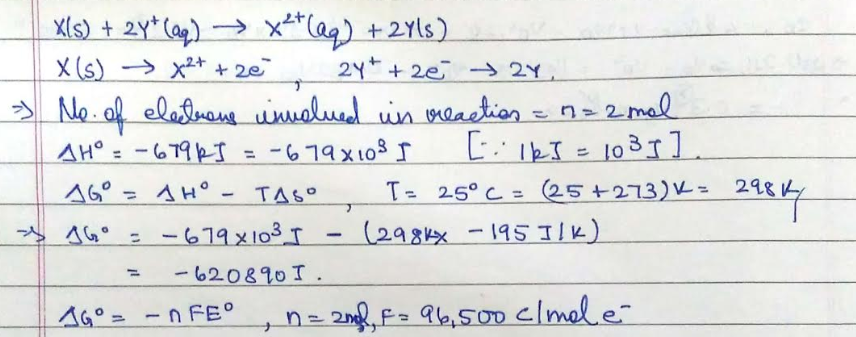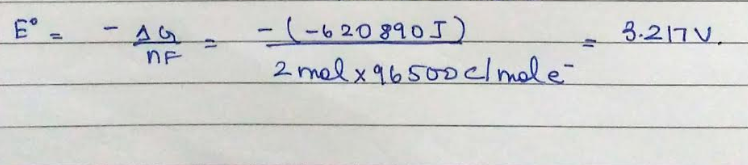#### Earn Coins

Coins can be redeemed for fabulous gifts.

Similar Homework Help Questions
• ### Free-energy change, AGº, is related to cell potential, Eº, by the equation AG° = -nFE° where...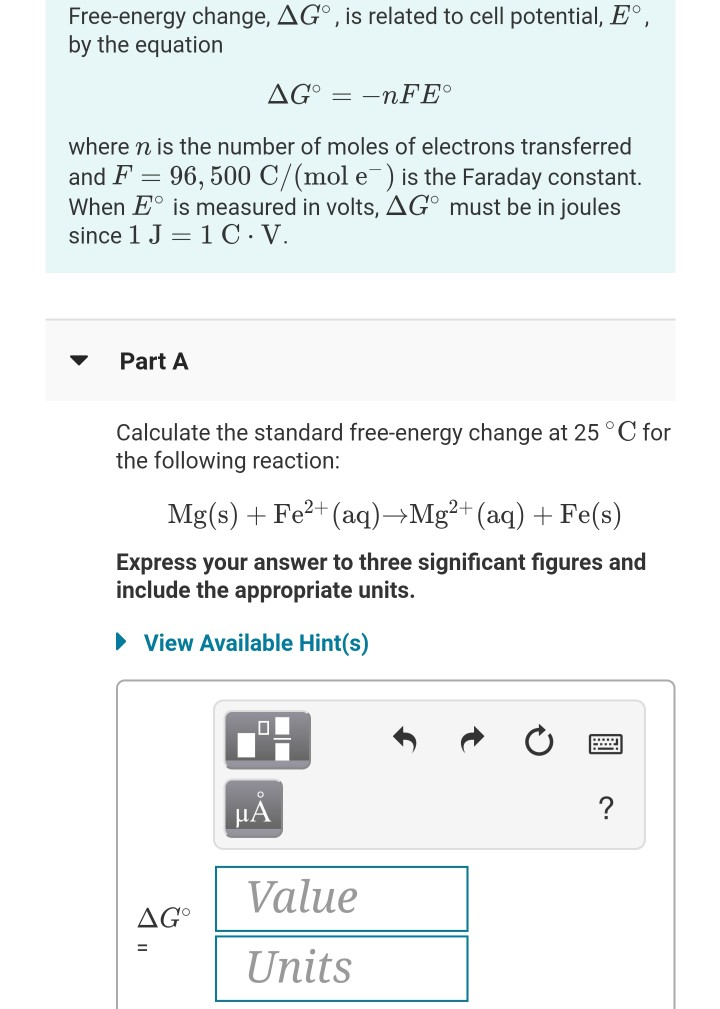Free-energy change, AGº, is related to cell potential, Eº, by the equation AG° = -nFE° where n is the number of moles of electrons transferred and F = 96,500 C/(mol e ) is the Faraday constant. When Eº is measured in volts, AGⓇ must be in joules since 1 J =1C.V. Part A Calculate the standard free-energy change at 25°C for the following reaction: Mg(s) + Fe2+ (aq)Mg2+ (aq) + Fe(s) Express your answer to three significant figures and include...

• ### Calculate the standard cell potential at 25 ∘C for the reaction X(s)+2Y+(aq)→X2+(aq)+2Y(s) where ΔH∘ = -627...

Calculate the standard cell potential at 25 ∘C for the reaction X(s)+2Y+(aq)→X2+(aq)+2Y(s) where ΔH∘ = -627 kJ and ΔS∘ = -153 J/K .

• ### Calculate the standard cell potential at 25 ∘C for the reaction X(s)+2Y+(aq)→X2+(aq)+2Y(s) where ΔH∘ = -795...

Calculate the standard cell potential at 25 ∘C for the reaction X(s)+2Y+(aq)→X2+(aq)+2Y(s) where ΔH∘ = -795 kJ and ΔS∘ = -349 J/K .

• ### Calculate the standard cell potential at 25 ∘C for the reaction X(s)+2Y+(aq)→X2+(aq)+2Y(s) where ΔH∘ = -829...

Calculate the standard cell potential at 25 ∘C for the reaction X(s)+2Y+(aq)→X2+(aq)+2Y(s) where ΔH∘ = -829 kJ and ΔS∘ = -367 J/K

• ### 9. Calculate the standard cell potential at 25 ∘C for the reaction X(s)+2Y+(aq)→X2+(aq)+2Y(s) where ΔH∘ =...

9. Calculate the standard cell potential at 25 ∘C for the reaction X(s)+2Y+(aq)→X2+(aq)+2Y(s) where ΔH∘ = -725 kJ and ΔS∘ = -289 J/K .

• ### Calculate the standard cell potential at 25 ∘C for the reaction X(s)+2Y+(aq)→X2+(aq)+2Y(s) where ΔH∘ = -923...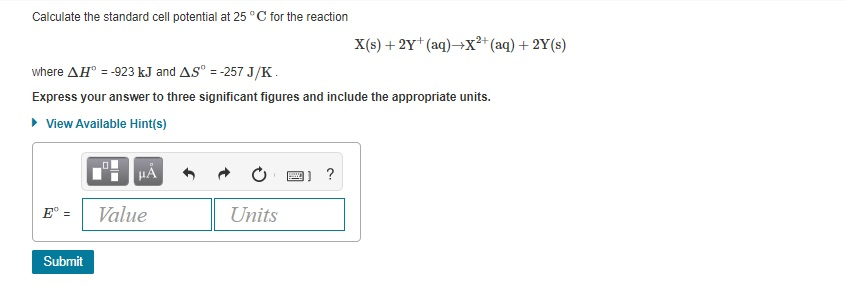Calculate the standard cell potential at 25 ∘C for the reaction X(s)+2Y+(aq)→X2+(aq)+2Y(s) where ΔH∘ = -923 kJ and ΔS∘ = -257 J/K . Express your answer to three significant figures and include the appropriate units. Calculate the standard cell potential at 25°C for the reaction X(s) +2Y+ (aq)+X2+ (aq) + 2Y(s) where AH = -923 kJ and AS = -257 J/K. Express your answer to three significant figures and include the appropriate units. View Available Hint(s) CO 2 ? E°...

• ### The equilibrium constant, K. for a redox reaction is related to the standard potential, E, by...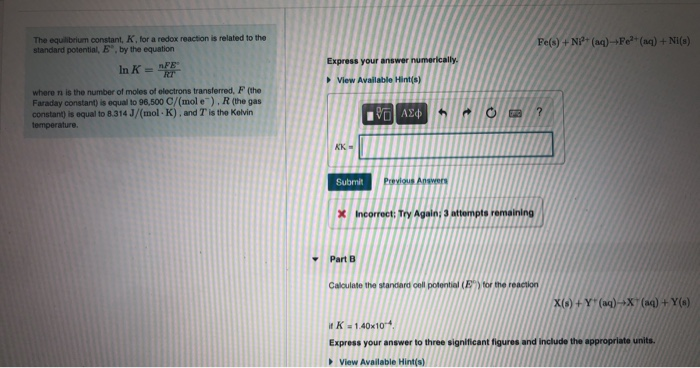The equilibrium constant, K. for a redox reaction is related to the standard potential, E, by the equation Fe(s) + Ni+ (aq) +Fe?+ (aq) + NI(s) FE In K = Express your answer numerically. View Available Hints) where n is the number of moles of electrons transferred, F (the Faraday constant) is equal to 96,500 C/(mole). R (the gas constant) is equal to 8.314 J/(mol-K). and T is the Kelvin temperature. ΟΙ ΑΣΦ h ? KK- Submit Previous Answers *...

• ### The equilibrium constant, K, for a redox reaction is related to the standard potential, Eº, by...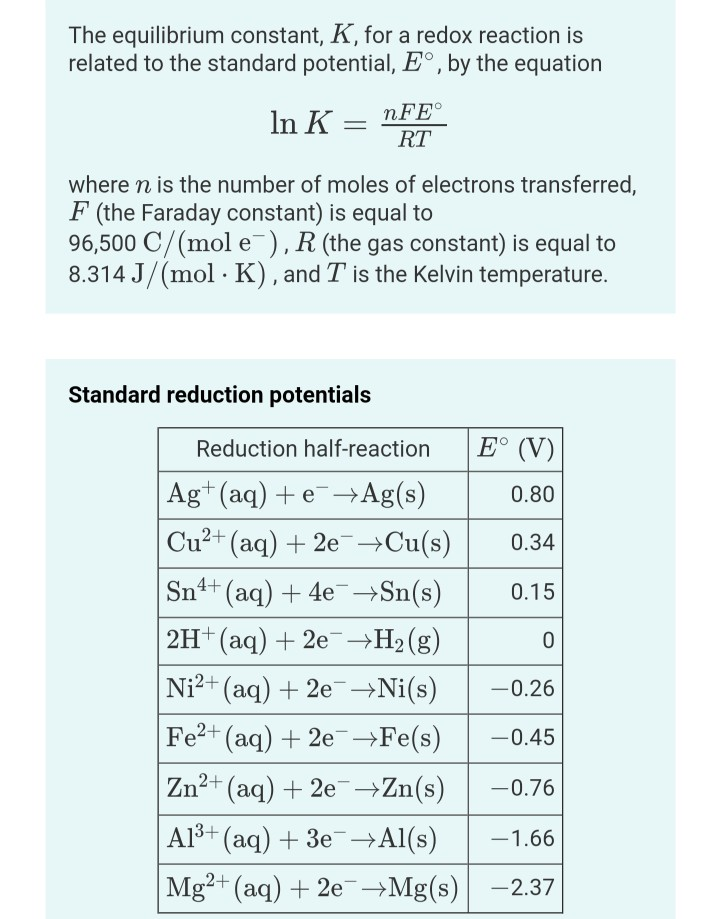The equilibrium constant, K, for a redox reaction is related to the standard potential, Eº, by the equation In K = nFE° RT where n is the number of moles of electrons transferred, F (the Faraday constant) is equal to 96,500 C/(mol e), R (the gas constant) is equal to 8.314 J/(mol · K), and T is the Kelvin temperature. Standard reduction potentials Reduction half-reaction E° (V) Ag+ (aq) + e +Ag(s) 0.80 Cu²+ (aq) + 2e + Cu(s) 0.34...

• ### Standard free energy change (Delta degree G) and cell potential (E degree) can be said to...Standard free energy change (Delta degree G) and cell potential (E degree) can be said to measure the same thing and are convertible by the equation: Delta G degree = nF E degree _cell where n is the total number of moles of electrons being transferred, and F is Faraday constant 9.6- 1853415 times 10^C/mol. The free energy (Delta G degree) of a spontaneous reaction is always negative. For each of the electrochemical cells below, calculate the free energy of...

• ### At equilibrium, the free energy change, ΔG, of any system is zero: ΔG=0=ΔH−TΔS So, under equilibrium...

At equilibrium, the free energy change, ΔG, of any system is zero: ΔG=0=ΔH−TΔS So, under equilibrium conditions, this equation can be rearranged as T=ΔHΔS Part A Glacial acetic acid is the concentrated form of acetic acid, the acid in vinegar. The term "glacial" refers to the appearance of the solid form, which resembles glacial ice. What is the melting point of this compound, in degrees Celsius, based on its thermodynamic data shown here? ΔHfus (kJ/mol) = 24.32 ΔSfus [J/(K⋅mol)] =...